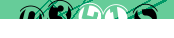# SQL: PostgreSQL SELECT

The most commonly used statement in SQL is the SELECT. Knowing how it works and some of the advanced options can significantly improve the performance of your application.

## DISTINCT, GROUP BY and HAVING

The SELECT DISTINCT statement by itself is useful for getting a list of unique values in a field:

`SELECT DISTINCT name FROM table;`

But in combination with GROUP BY becomes extremely useful. With this command you can list not only the unique values, but the number of times each occurs:

`SELECT DISTINCT name, COUNT(*) AS count FROM table GROUP BY name ORDER BY COUNT(*);`

Perhaps more practical example is the following:

`SELECT DISTINCT SUBSTR(lastname, 1, 1), COUNT(*) FROM table GROUP BY SUBSTR(lastname, 1, 1);`

Finally, we can limit the output to show only the top (or bottom) of the list:

`SELECT DISTINCT name, COUNT(*) AS count FROM table GROUP BY name HAVING COUNT(*)>=10 ORDER BY COUNT(*);`

## OFFSET, LIMIT and RANDOM

To limit results to the first limit rows:

`SELECT * FROM TABLE LIMIT limit;`

To limit results to limit rows, skipping the first offset rows:

`SELECT * FROM TABLE OFFSET \$offset LIMIT limit;`

To make a random selection of limit rows:

`SELECT * FROM TABLE ORDER BY RANDOM() LIMIT limit;`

Note: If you have a lot of data then this can put unnecessary load on the database - a random value needs to be generated for each record. In this situation you might be better off generating random numbers in PHP and using OFFSET and LIMIT to select just those rows.

## INTERVAL

Quite often you want to SELECT (or DELETE) records that are 'newer' or 'older' than a certain interval:

```SELECT COUNT(*) AS count FROM table WHERE timestamp < NOW() - INTERVAL '1 month'; SELECT COUNT(*) AS count FROM table WHERE timestamp > NOW() + INTERVAL '2 weeks';```

The units used in the INTERVAL can be "second, minute, hour, day, week, month, year, decade, century, millennium, or abbreviations or plurals of these units". See the link to the documentation under References for more information.

## CASE Statement

The SQL CASE statement can very useful in tight situations. In this example it's used to avoid division by zero errors when calculating percentages:

`SELECT id, name, CASE WHEN (yes+no)>0 THEN 100 * yes/(yes+no) ELSE 0 END AS percent`

If yes and no (both integers) are zero then the value for percent will be zero. If one or both are non-zero then the return value will be the percentage: yes/(yes + no).

## References

< SQL

#### Send a message to The Art of Web: